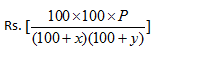# Short Tricks for Profit and Loss Maths

Short Tricks for Profit and Loss and Important question Profit and Loss

Marked Price Formula (MP)

Discount = Marked Price – Selling Price
And Discount Percentage = (Discount/Marked price) x 100

Profit and Loss Formulas

Profit or Gain = Selling price – Cost Price
Loss = Cost Price – Selling Price

Profit percentage = (Profit /Cost Price) x 100
Loss percentage = (Loss / Cost price) x 100

### Short Tricks for Profit and Loss formulas

1. One can generate a profit only if Selling Price> Cost Price

2. One generates a loss when Selling Price < Cost Price.

3. Profit = Selling Price – Cost Price
%profit = {(Selling Price – Cost Price)/Cost Price} x 100

4. Loss = Cost Price – Selling Price
%Loss = {(Cost Price – Selling Price)/Cost Price} x 100

5. Sale price :- If there is a profit of P %,
Cost Price = C
Then SP = {(100+P)/100}xC

6. If there is a loss of L %,
Cost Price = C
Then
SP = {(100-L)/100}xC

7. Cost price :-
If there is a profit of P %,
Cost Price = C
Sale price= SP
Then C = {100/(100+p)} x SP
If there is a loss of L %,
Then
C = {100/(100-L)}xSP

8. A dishonest dealer claims to sell his goods at cost price ,but he uses a weight of lesser weight .Find his gain%.

9. A shopkeeper sells an item at a profit of x % and uses a weight which is y % less .find his total profit

10. When dealer sells goods at loss on cost price but uses less weight .

11. A dishonest dealer sells goods at x % loss on cost price but uses a gm instead of b gm . his profit or loss percent :-

Note :- profit or loss will be decided according to sign .if +ive it is profit ,if –ve it is loss .

12.  If the price of an item increases by r%  , then the reduction in consumption so that  expenditure remains the same  ,is

13. If the price of a commodity decreases by r% then increase in consumption , so as not to decrease expenditure on this item is

14. A reduction of x% in price enables a person to buy y kg more for Rs. A. Then the

15. When there are two successive profits of x% and y% then the net percentage profit =[x+y+xy/100]

When there is a profit of x% and loss of y% then net percentage profit or loss = [x – y – xy/100]

Note: If the final sign in the above expression is positive then there is net profit but if it is negative then there is net loss.

16. A sells goods to B at a profit of x% andB sells it to C at a profit of y%. If C pays RsP for it,then the cost price for A isNote:- for loss replace plus sign with minus .

17. When each of the two things is sold at the same price,and a profit of p% is made on the first and a loss of L% is made on the second,then the percentage gain or loss is .

18. If profit percentage and loss percentage are equal, put P=L

=>   %loss = p2 /100

19.

Q. 1: Suppose a shopkeeper has bought 1 kg of apples for 100 rs. And sold it for Rs. 120 per kg. How much is the profit gained by him?

Solution:
Cost Price for apples is 100 rs.
Selling Price for apples is 120 rs.
Then profit gained by shopkeeper is ; P = SP – CP
P = 120 – 100 = Rs. 20/-

Q.2: For the above example calculate the percentage of the profit gained by the shopkeeper.

Solution:
We know, Profit percentage = (Profit /Cost Price) x 100
Therefore, Profit percentage = (20/100) x 100 = 20%.

Q.3: A man buys a fan for Rs. 1000 and sells it at a loss of 15%. What is the selling price of the fan?

Solution:
Cost Price of the fan is Rs.1000
Loss percentage is 15%
As we know, Loss percentage = (Loss/Cost Price) x 100
15 = (Loss/1000) x 100
Therefore, Loss = 150 rs.
As we know,
Loss = Cost Price – Selling Price
So, Selling Price = Cost Price – Loss
= 1000 – 150
Selling Price = R.850/-

Q.4: If a pen cost Rs.50 after 10% discount, then what is the actual price or marked price of the pen?

Solution:
MP x (100 – 10) /100 = 50
MP x (90/100) = 50
MP = (50 x 100)/90
MP = Rs. 55.55/-